• Call Now

1800-102-2727•

# Boyle's law - Definition, formula, some real life examples, Practice problems, FAQs

Each of us has encountered a syringe when visiting a doctor. It is a medical tool used for fluid injection or withdrawal. It is made up of a sliding plunger attached to a hollow barrel. A syringe functions similarly to a reciprocating pump. The fluid will inject when the plunger is pressed, and it will withdraw when the plunger is pulled.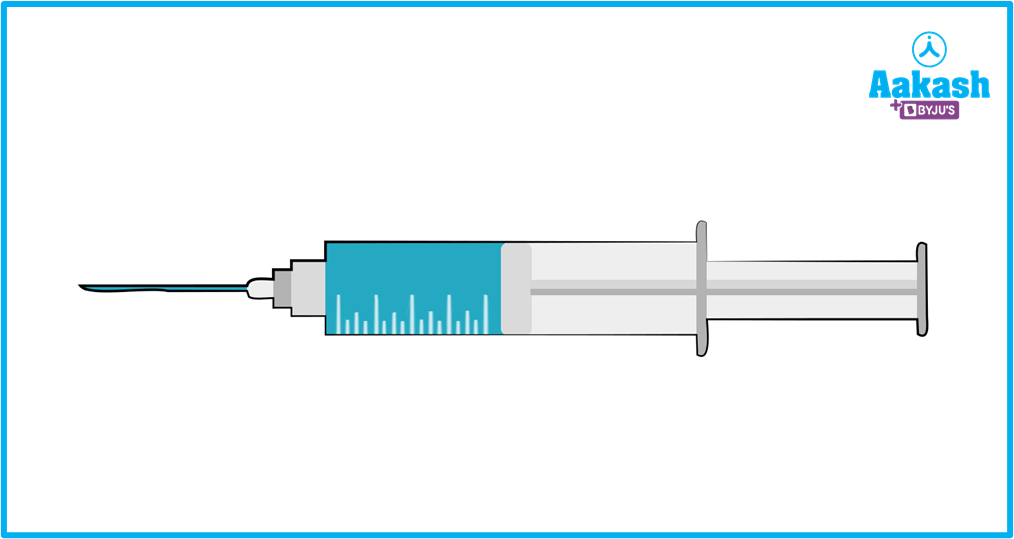Do you think, what is happening? The amount of fluid in the barrel decreases as the plunger is pushed. Due to a momentary increase in pressure brought on by the volume reduction, the fluid is then pushed into the patient's body. Similar to this, pulling the plunger expands the fluid's volume. The fluid's pressure briefly drops as a result, and external fluid is extracted. This can be explained by the boyle's law. Let's learn what Boyle's law is !

Table of content

• Boyle's law
• Definition and formula for Boyle's law
• Some real life example of Boyle’s law
• Practice problems
• FAQs

## Boyle's law

According to the law, as long as the temperature of a closed system doesn't vary, the absolute pressure and volume of a given amount of confined gas are inversely related. At constant mass and temperature, the volume-pressure relationship for a gas can be mathematically described as follows.

$P\propto \frac{1}{V}$

Where, P is the pressure the gas is exerting, and V is the volume it is occupying. By including the constant k, this proportionality can be transformed into an equation as,

$P=\frac{k}{V}$

PV=k

Hence Boyle's law states that if a gas's pressure doubles while its temperature remains constant, its volume will also be reduced by half. The cause is the intermolecular force that exists between the molecules of the gaseous substance. A gaseous substance occupies more area in a container when it is free due to the dispersed nature of the molecules.

The graph below shows the pressure v/s volume curve for a set mass of gas kept at a constant temperature.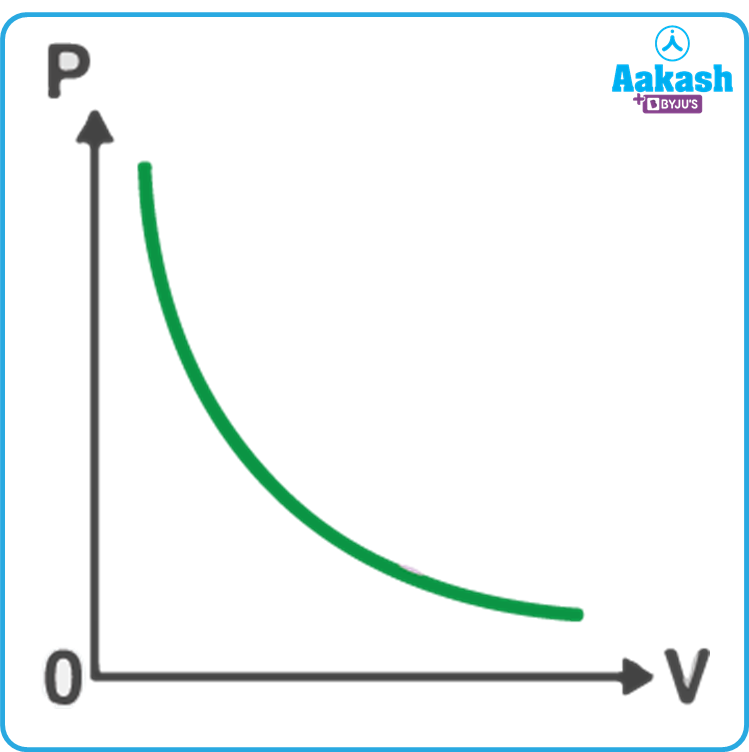If we draw a graph between the pressure and the reciprocal of the volume, then it will be straight line as shown in figure below,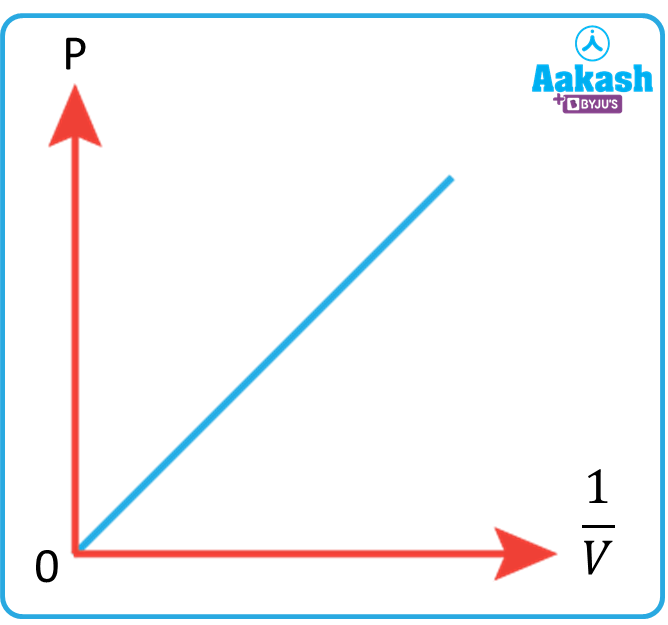## Definition and formula for Boyle's Law

According to Boyle's law, a gas's pressure will change whenever its volume changes, given its quantity and temperature remain constant. In other words, the product of a gas's initial pressure to its initial volume is equal to the product of gas's final pressure to that final volume (at constant temperature and number of moles). If P1, V1 is the initial pressure and volume of the of the system and P2, V2 is the final pressure and volume of the system, then this law has the following mathematical formulation:

${P}_{1}{V}_{1}={P}_{2}{V}_{2}$

Or $\frac{{P}_{1}}{{P}_{2}}=\frac{{V}_{2}}{{V}_{1}}$

When a gas's container's volume is reduced, this equation can be used to determine how much pressure will increase on the container's walls (when its quantity and absolute temperature remain unchanged).

## Some real life examples of Boyle’s law

Boyle's law describes how a gas's pressure and volume are related. Robert Boyle made the first discovery in the seventeenth century. He discovered that, given a fixed quantity of gas, the pressure of a gas is inversely proportional to its volume at constant temperature. Following are some examples or applications from real-world situations.

1. Syringe - A syringe is a piece of medical equipment used for injecting or withdrawing fluids. A cylinder holds the fluid inside, and a plunger changes the pressure. As the plunger is lowered, the fluid's volume reduces, raising the pressure. Similar to this, as the plunger is raised, the volume rises but the pressure falls.
1. Human lungs - An essential body organ is the lungs. They are crucial components of the respiratory system. There is a small drop in pressure when the lungs enlarge. Therefore, the body's internal pressure is lower than its external pressure. As a result, external air enters the body. This process is known as inhalation. The volume of the lungs reduces as they relax, temporarily raising pressure in relation to the outside. The body then exhales the air.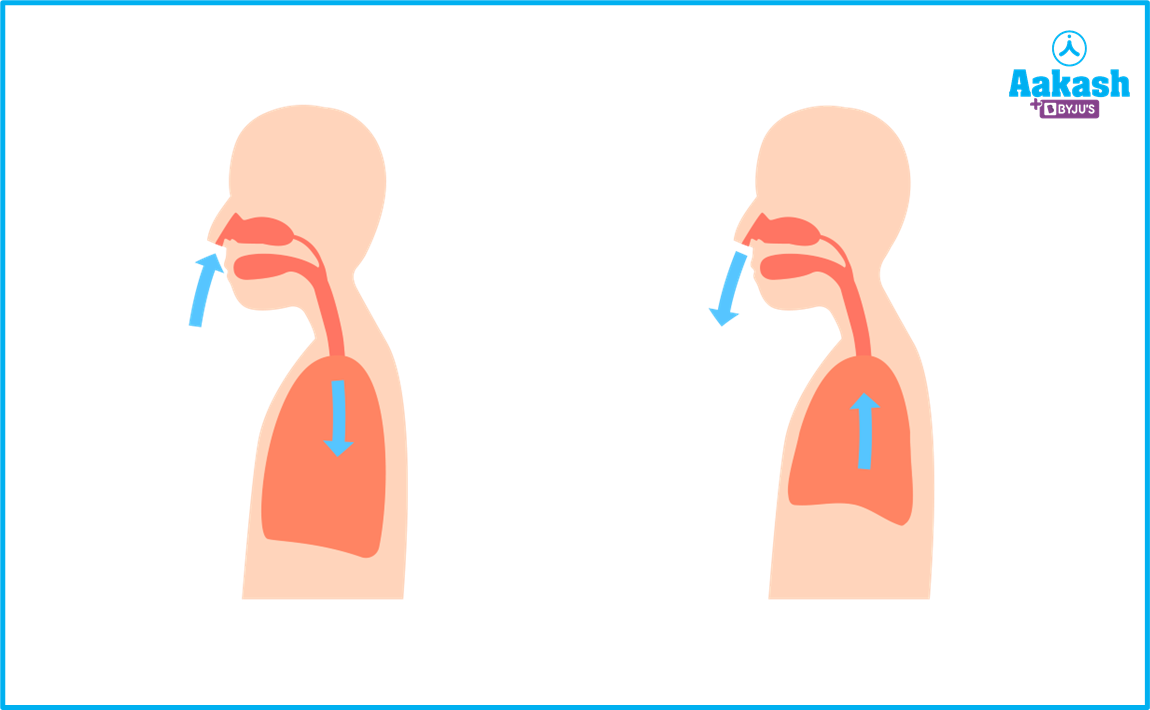1. Bicycle pump - A syringe and a hand bicycle pump have similar functions. The pressure inside a pump will momentarily rise when the handle is depressed. To put it another way, the gas inside is confined. The effect is that the pressured gas is driven into a vehicle's tyre.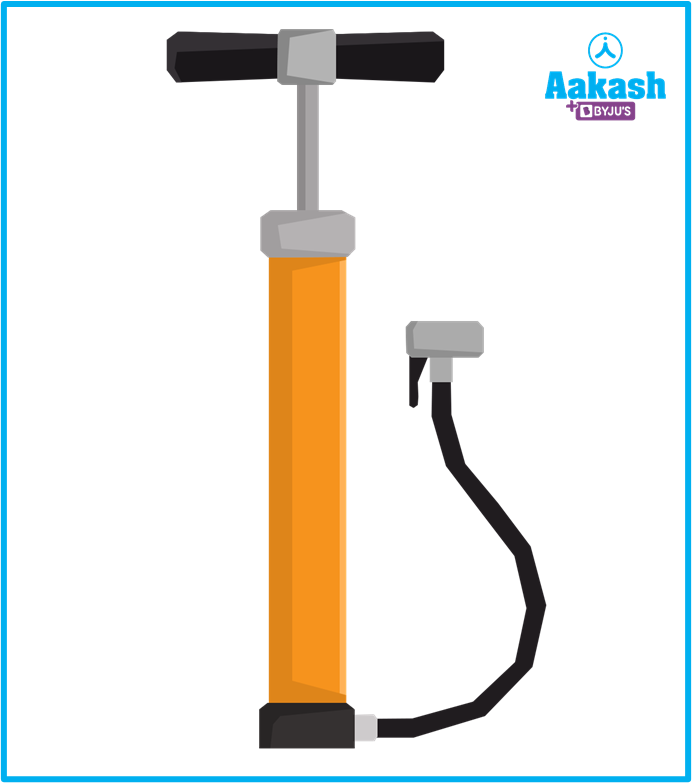1. Air bubble - In water, air bubbles enlarge as they rise. The liquid's surrounding pressure lowers as the bubbles rise. And the air bubbles grow in size in accordance with Boyle's law.1. Spray paint - Boyle's law is the basis for how spray paints function. On the body of the can it is confined in, the paint molecules exert a substantial amount of pressure. When the can's top is depressed, the volume inside the can decreases and the paint is ejected under strong pressure.

## Practice problems

Q1. At room temperature, hydrogen is inflated into a balloon. If the pressure goes over 1.3 bar, it will burst. What volume may the balloon be expanded to if the gas has a 3.45 L volume at 1 bar pressure?

And P2=1.3 bar

According to the Boyle’s law,

${P}_{1}{V}_{1}={P}_{2}{V}_{2}$

$1×3.45=1.3×{V}_{2}$

V2=2.6538 L

The volume of the balloon is 2.6538 L.

Q2. A specific amount of gas is present in a vessel with a 150 mL capacity at 1.5 bar pressure and 28 °C. The gas is then transferred to a 200 mL vessel at 28 °C. What pressure would it have?

Answer. Given r and V1=150 mL

And V2=200 ml

According to the Boyles’ law,

${P}_{1}{V}_{1}={P}_{2}{V}_{2}$

P2=1.125 bar

The pressure of the gas is 1.125 bar

Q3. A gas takes up 20 liters at 37 mm Hg of pressure. When the pressure is raised to 50.0 mm Hg, what is the volume?

A. Given P1=37 mm Hg and V1=20 liters

And P2=50.0 mm Hg

According to the Boyles’ law,

${P}_{1}{V}_{1}={P}_{2}{V}_{2}$

$37×20=50×{V}_{2}$

V2=14.8 liters

The volume of the gas is 14.8 liters.

Q4. What pressure does an atmospheric gas need to be compressed to in order to fit a 400.0 cubic feet of gas into a 3 cubic foot tank?

Answer. Given P1=1 atm and V1=400.0 cubic feet

And V2=3 cubic foot

According to the Boyles’ law,

${P}_{1}{V}_{1}={P}_{2}{V}_{2}$

$1×400={P}_{2}×3$

P2=133.33 atm

Hence the pressure needed is 133.33 atm.

## FAQs

Q1.Under what circumstances Boyle's law is relevant?
In an isothermal process where the temperature stays constant, Boyle's law is applicable.

Q2. Is Boyle's law applicable to liquids?
Liquids cannot be compressed, like gasses, because their particles are already relatively closely spaced. So Boyle's law is not applicable to liquids.

Q3. Why does Boyle's law not apply in high pressure situations?
Answer: Boyle's law only holds true at low pressure because at high pressure, gases do not behave like ideal gasses.

Q4. How does Boyle's law operate?
Answer: According to Boyle's law, the relationship between a gas's pressure and volume is inverse at a given temperature. I.e. when the temperature remains the same, pressure rises as volume decreases and vice versa.Talk to our expert
Resend OTP Timer =
By submitting up, I agree to receive all the Whatsapp communication on my registered number and Aakash terms and conditions and privacy policy